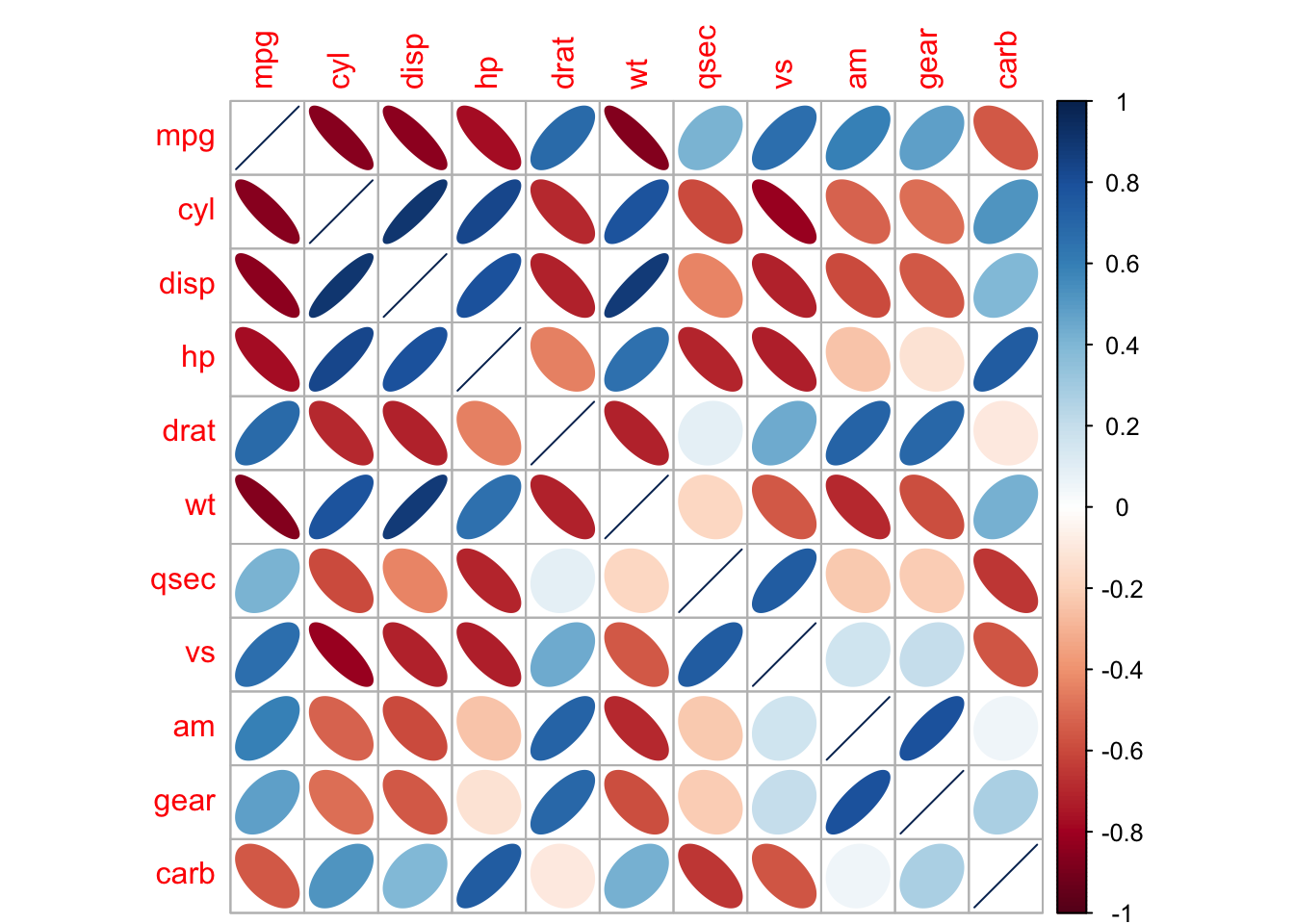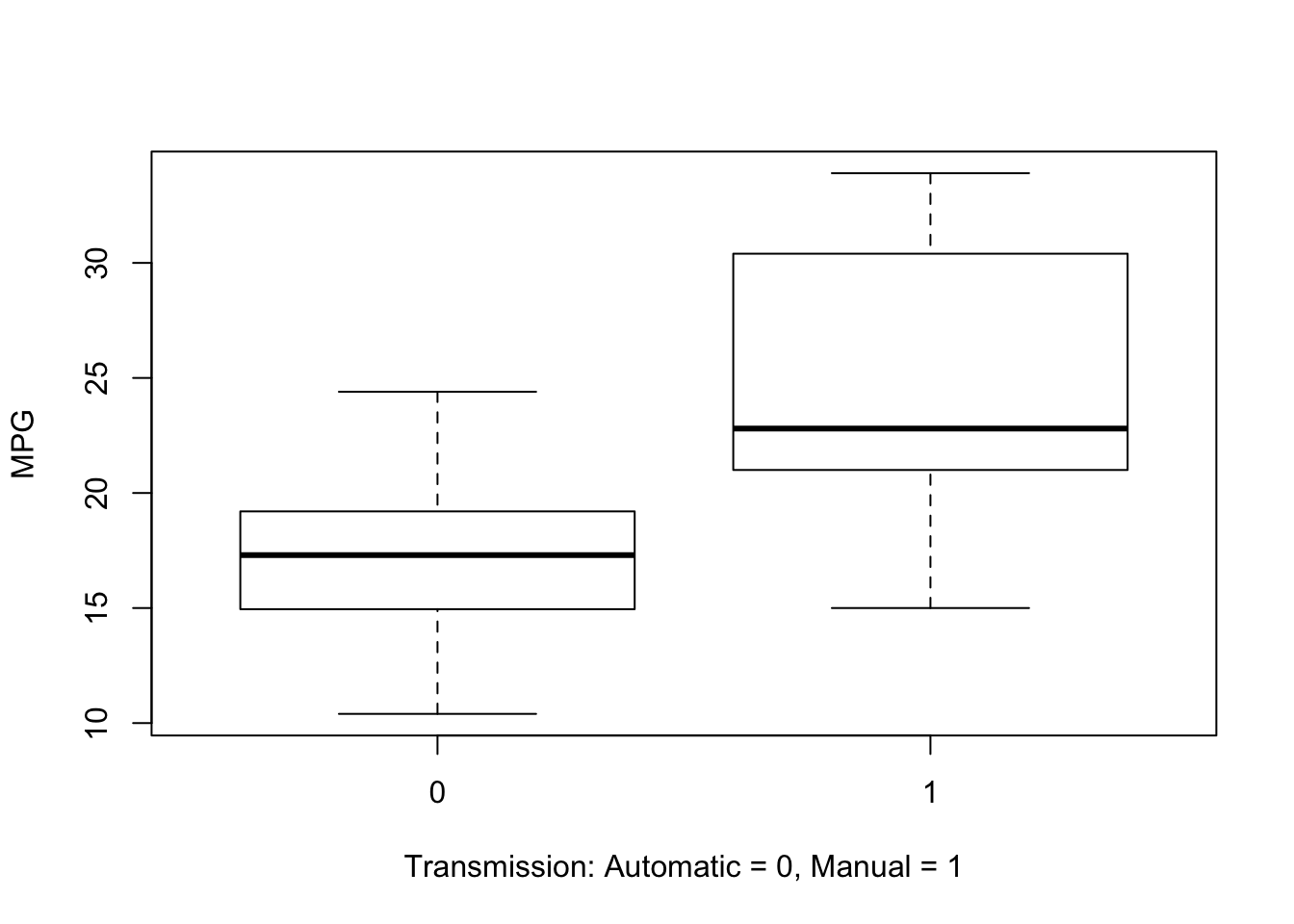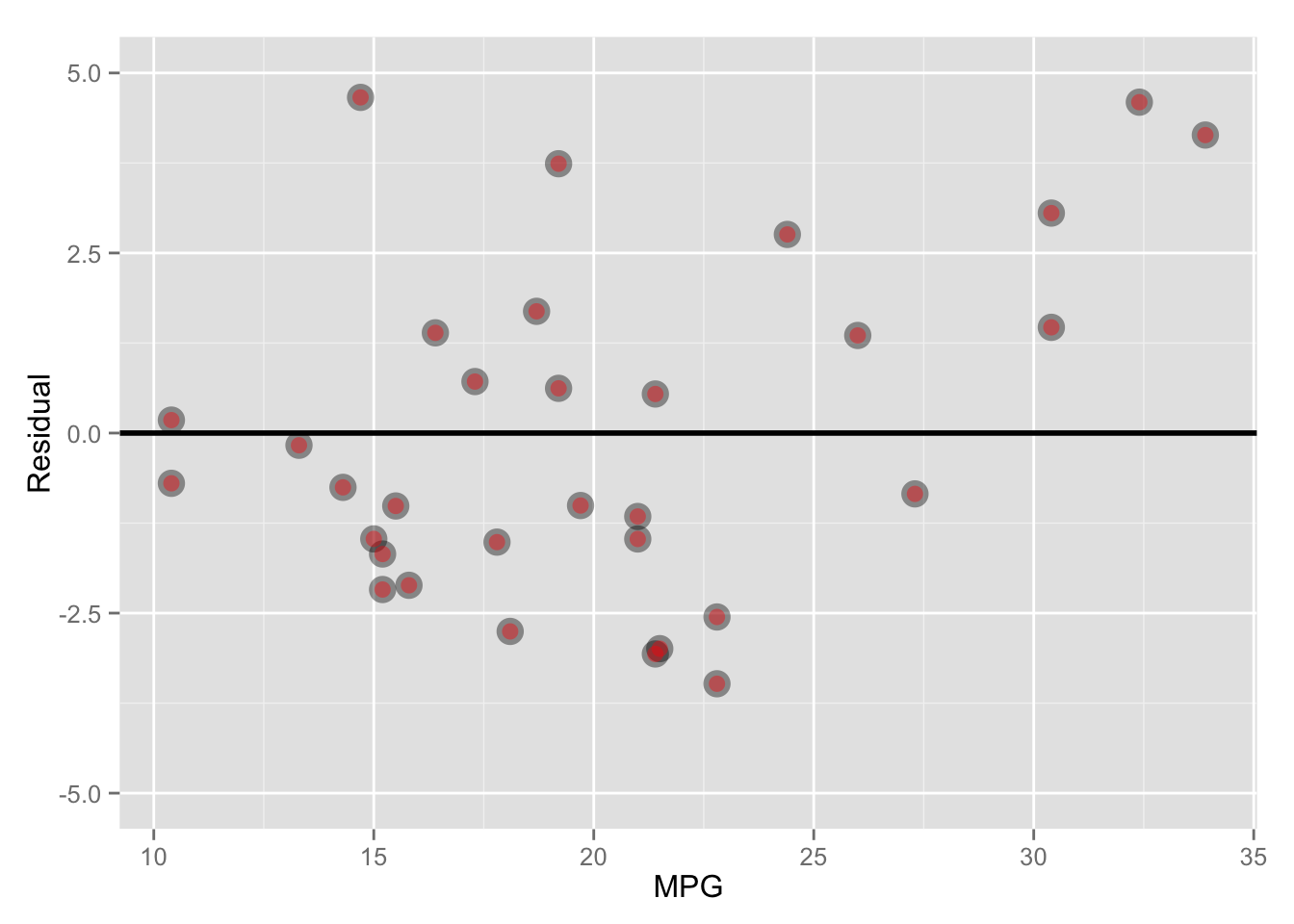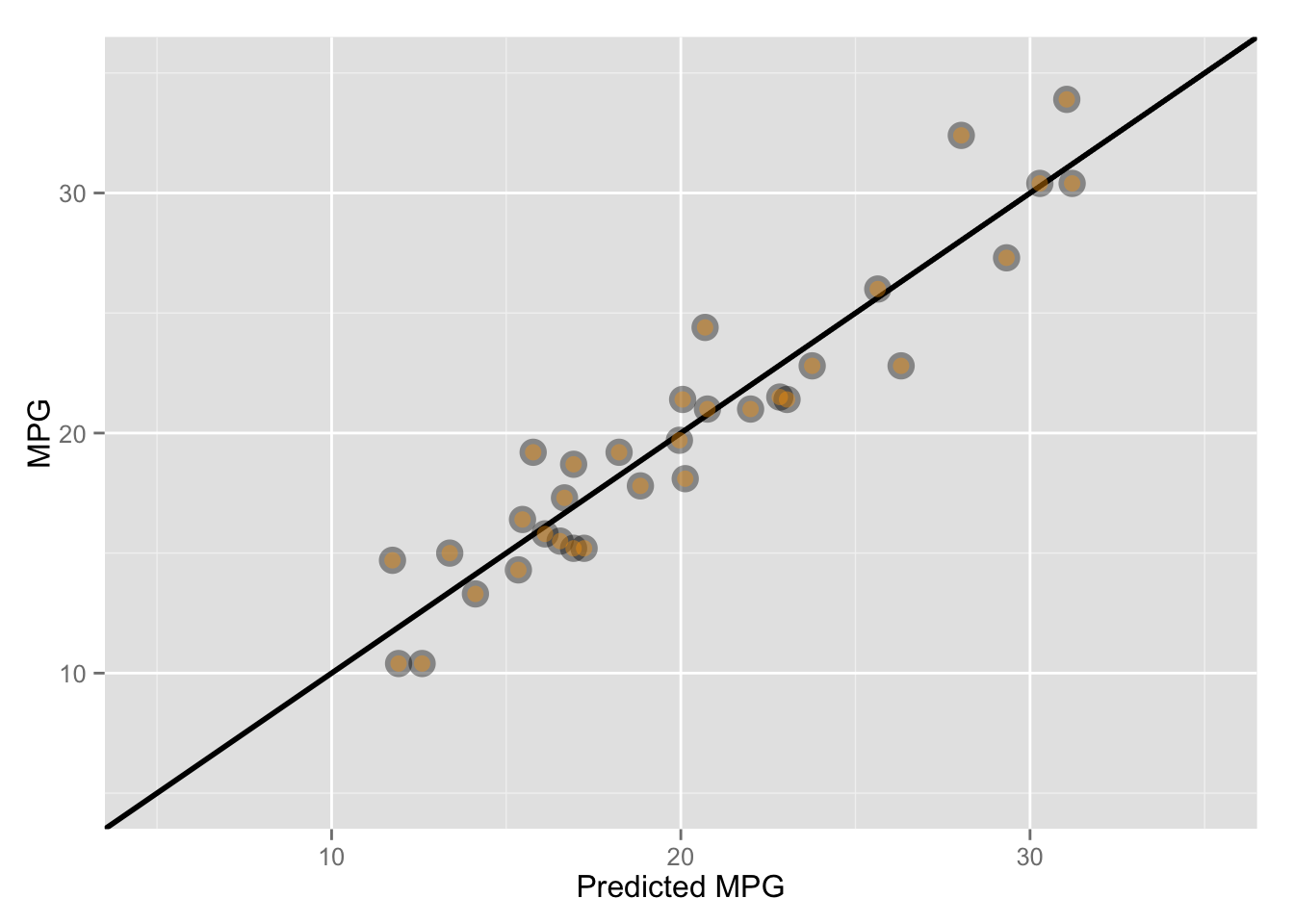## Executive Summary

Using data extracted from the 1974 Motor Trend US magazine (mtcars dataset), we aimed to find whether the transmission type of the car (manual or automatic) had a statistically significant impact on the fuel efficiency of a car, as measured in miles per gallon (MPG). Through a series of linear regression models and other tests, we found strong evidence to suggest that transmission type does have a statically significant impact on the MPG for the cars analyzed. An initial linear model suggested that manual transmission improves MPG by 2.936 once other variables are controlled for. However, adding an interaction term suggested that the improvement in MPG is dependent on the weight of the car. The final model indicated that the MPG improvement offered by a manual transmission is given by the equation 14.079 - 4.141 * wt where wt is the weight of the car in lb/1000.

## Exploratory Analysis

Looking at the correlations between the variables in the dataset (Figure 1), we see that there is a strong negative relationship between several variables and MPG. This includes the number of cylinders (cyl), the engine displacement (disp), horsepower (hp) and weight (wt). We also see hp is strongly correlated with the disp and cyl, suggesting increasing hp leads to decreasing MPG. 1/4 mile time (qsec) also has a strong negative correlation with hp.

Finally, looking at correlation between MPG and the automatic/manual indicator (am), there is a positive correlation of almost exactly 0.6. Using a box plot (Figure 2) we also see there is a significant difference in the distributions of cars when split by am. Both these pieces of evidence suggest that manual cars are more fuel efficient than automatics.

## Using Stepwise Regression to Select a Model

In order to provide stronger support for the hypothesis that manual cars are more fuel efficient, we used linear regression to construct models that can assess the impact of am on mpg, holding the other variables constant. The first step in building the linear model is variable selection. To do this we used stepwise regression. This algorithm can be used in several ways, and with different measures of model quality.

After testing the various combinations of methods and quality measures, the best results (in terms of adjusted R-squared, the significance of the predictors and model simplicity) were returned using the Bayesian Information Criterion (BIC) method to assess model quality, and either the “backwards” or “both” methodology (starting from a model with all the variables included).

``````fit.full <- lm(mpg ~ factor(cyl) + disp + hp + drat + wt + qsec + factor(vs) + factor(am) + factor(gear) + factor(carb), mtcars)
fit.step.BIC <- step(fit.full, direction = "both", trace = 0, k = log(nrow(mtcars))) # BIC
coef(fit.step.BIC)``````
``````## (Intercept)          wt        qsec factor(am)1
##    9.617781   -3.916504    1.225886    2.935837``````

The three variables selected to be used in this model were wt, qsec and am. The adjusted R-squared for the model is 0.8336, and the three variable coefficients are all significant at the 5% confidence level. The intercept however is not significant. Looking at the plot of the residuals for this model (Figure 3), we see no significant indications of poor model fit or heteroskedasticity.

The coefficient for am suggests that having a manual car adds 2.936 MPG above that of an automatic (5% confidence interval of 0.046 to 5.826 MPG) with weight and the 1/4 mile time held constant. The model also suggests that a 1,000lb increase in weight leads to a 3.917 reduction in MPG, while a 1 second increase in 1/4 time (implying a less powerful engine) increases MPG by 1.226.

Going back to Figure 1, there is reason to believe there may be an interaction between the wt and am variables. We tested for this by building a model with an interaction term.

``````fit.interaction <- lm(mpg ~ wt + qsec + factor(am) + factor(am):wt, mtcars)
coef(fit.interaction)``````
``````##    (Intercept)             wt           qsec    factor(am)1 wt:factor(am)1
##       9.723053      -2.936531       1.016974      14.079428      -4.141376``````

The results of the model show the interaction between am and wt is significant, and its inclusion improves the overall fit (Figure 4). The adjusted R-squared increased from 83.36% to 88.04%. All the coefficients (excluding the intercept) are now also significant at the 1% level. Again looking at the plot of the residuals (Figure 5), we see no indications of poor model fit or heteroskedasticity.

Adding the interaction term has also modified the coefficients for our original terms, with the coefficient for am now significantly higher at 14.08 (5% confidence interval of 7.031 to 21.128). However, a coefficient of -4.141 for the interaction term suggests the MPG gain in changing from auto to manual now decreases with increasing wt. When the weight of the car is zero (wt = 0), the wt:am term cancels out and the estimated gain is 14.08 MPG. For a car with a weight of 1,000lb (wt = 1), the estimated gain reduces to 9.94 MPG. Continuing this, we can plot the estimated gain in MPG against wt (see Figure 6).

## Appendix

### Figure 1 - Correlations Between All Variables### Figure 2 - Box Plot of MPG vs. Transmission### Figure 3 - Stepwise Model - Residuals vs. MPG### Figure 4 - Model with Interaction Term - Actual MPG vs. Predicted MPG